# Gamma function facts for kids

Kids Encyclopedia Facts

In mathematics, the gamma function (Γ(z)) is an extension of the factorial function to positive real and complex numbers. The argument of the function is shifted down by one. This means that if n is a positive integer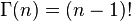$\Gamma(n) = (n-1)!\,$

The gamma function is defined for all complex numbers. But it is not defined for negative numbers and zero. For a complex number whose real part is positive, it is defined by: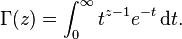$\Gamma(z) = \int_0^\infty t^{z-1} e^{-t}\,{\rm d}t.$

This improper integral is convergent. Legendre first used the notation Γ(z). If the real part of the complex number z is positive (Re(z) > 0), then the integral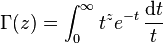$\Gamma(z) = \int_0^\infty t^{z} e^{-t}\,\frac{{\rm d}t}{t}$

converges absolutely. It is known as the Euler integral of the second kind. If we use integration by parts, we see that the functional equation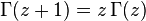$\Gamma(z+1)=z \, \Gamma(z)$ holds true for the gamma function.

We see that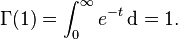$\Gamma(1) = \int_0^\infty e^{-t}\,{\rm d} = 1.$ So, by the recursive relation$\Gamma(z+1)=z \, \Gamma(z)$, we get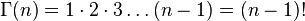$\Gamma(n) = 1 \cdot 2 \cdot 3 \dots (n-1) = (n-1)!\,$

for all positive integers n.

## Properties

### General

Euler's reflection formula is an important functional equation for the gamma function. It says: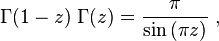$\Gamma(1-z) \; \Gamma(z) = {\pi \over \sin{(\pi z)}} ~,$

This means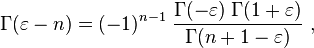$\Gamma(\varepsilon - n) = (-1)^{n-1} \; {{\Gamma(-\varepsilon) \; \Gamma(1+\varepsilon)} \over {\Gamma(n+1-\varepsilon)}} ~,$

The gamma function also has a duplication formula: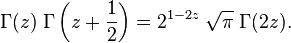$\Gamma(z) \; \Gamma\left(z + \frac{1}{2}\right) = 2^{1-2z} \; \sqrt{\pi} \; \Gamma(2z). \,$

This formula is a special case of the multiplication theorem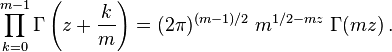$\prod_{k=0}^{m-1}\Gamma\left(z + \frac{k}{m}\right) = (2 \pi)^{(m-1)/2} \; m^{1/2 - mz} \; \Gamma(mz)\,.$

A property of the function is that: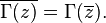$\overline{\Gamma(z)} = \Gamma(\overline{z}). \,\!$

A famous value of the function at a non-integral point is: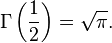$\Gamma\left(\frac{1}{2}\right)=\sqrt{\pi}. \,\!$

This is found if we set z = 1/2 in the reflection or duplication formulas. If we simply make the substitution u = √t in the integral definition of the gamma function, we get a Gaussian integral. In general, for non-negative integer values of n we have: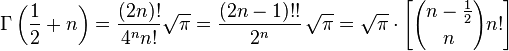$\Gamma\left(\frac{1}{2}+n\right) = {(2n)! \over 4^n n!} \sqrt{\pi} = \frac{(2n-1)!!}{2^n}\, \sqrt{\pi} = \sqrt{\pi} \cdot \left[ {n-\frac{1}{2}\choose n} n! \right]$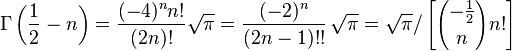$\Gamma\left(\frac{1}{2}-n\right) = {(-4)^n n! \over (2n)!} \sqrt{\pi} = \frac{(-2)^n}{(2n-1)!!}\, \sqrt{\pi} = \sqrt{\pi} / \left[ {-\frac{1}{2} \choose n} n! \right]$

where n!! denotes the double factorial and, when n = 0, (-1)!! = 1.

The derivatives of the gamma function are described in terms of the polygamma function. For example: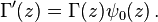$\Gamma'(z)=\Gamma(z)\psi_0(z)\,.$

For positive integer m the derivative of gamma function can be calculated as follows (here γ is the Euler–Mascheroni constant):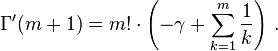$\Gamma'(m+1) = m!\cdot\left( - \gamma + \sum_{k=1}^m\frac{1}{k} \right)\,.$

The n-th derivative of the gamma function is: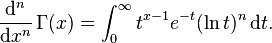$\frac{{\rm d}^n}{{\rm d}x^n}\,\Gamma(x) = \int_0^\infty t^{x-1} e^{-t} (\ln t)^{n} \,{\rm d}t.$

### Particular values

Some particular values of the gamma function are: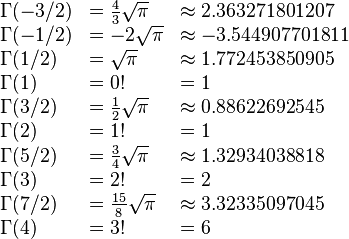$\begin{array}{lll} \Gamma(-3/2) &= \tfrac{4}{3} \sqrt{\pi} &\approx 2.363271801207 \\ \Gamma(-1/2) &= -2\sqrt{\pi} &\approx -3.544907701811 \\ \Gamma(1/2) &= \sqrt{\pi} &\approx 1.772453850905 \\ \Gamma(1) &= 0! &= 1 \\ \Gamma(3/2) &= \tfrac{1}{2}\sqrt{\pi} &\approx 0.88622692545 \\ \Gamma(2) &= 1! &= 1 \\ \Gamma(5/2) &= \tfrac{3}{4}\sqrt{\pi} &\approx 1.32934038818 \\ \Gamma(3) &= 2! &= 2 \\ \Gamma(7/2) &= \tfrac{15}{8}\sqrt{\pi} &\approx 3.32335097045\\ \Gamma(4) &= 3! &= 6 \\ \end{array}$

### Pi function

Gauss introduced the Pi function. This is another way of denoting the gamma function. In terms of the gamma function, the Pi function is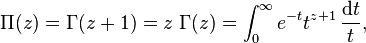$\Pi(z) = \Gamma(z+1) = z \; \Gamma(z) = \int_0^\infty e^{-t} t^{z+1}\,\frac{{\rm d}t}{t},$

so that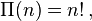$\Pi(n) = n!\,,$

for every non-negative integer n.

## Applications

### Analytic number theory

The gamma function is used to study the Riemann zeta function. A property of the Riemann zeta function is its functional equation: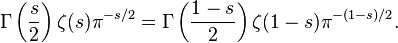$\Gamma\left(\frac{s}{2}\right)\zeta(s)\pi^{-s/2} = \Gamma\left(\frac{1-s}{2}\right)\zeta(1-s)\pi^{-(1-s)/2}.$

Bernhard Riemann found a relation between these two functions. This was in 1859 paper "Über die Anzahl der Primzahlen unter einer gegebenen Grösse" ("On the Number of Prime Numbers less than a Given Quantity")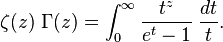$\zeta(z) \; \Gamma(z) = \int_0^\infty \frac{t^{z}}{e^t-1} \; \frac{dt}{t}.$

## Images for kidsGamma function Facts for Kids. Kiddle Encyclopedia.